Download PDF文章快速检索 高级检索

1. 江西理工大学信息工程学院, 江西赣州 341000;
2. 华南理工大学计算机与工程学院, 广东广州 510006;
3. 中山大学数据科学与计算机学院, 广东广州 510006

Advances in theoretical research of ant colony optimization
XIA Xiaoyun1,2, ZHOU Yuren31. School of Information Engineering, Jiangxi University of Science and Technology, Ganzhou 341000, China;
2. School of Computer Science and Engineering, South China University of Technology, Guangzhou 510006, China;
3. School of Data and Computer Science, Sun Yat-Sen University, Guangzhou 510006, China
Abstract: Theoretical investigations of the ant colony optimization (ACO) algorithm can help to improve our understanding of the theoretical basis of the algorithm and guide its appropriate application. Theoretical research on ACO has included analyses of early convergence, time complexity, and approximation performance. Investigation objectives have ranged from simple Boolean functions, to combinatorial optimization and practical application problems, to the analysis of NP-hardness problems. In this paper, we survey state-of-the-art theoretical ACO research from two aspects: the most common mathematical techniques and those less common. In addition, we introduce two mathematical analysis tools, including fitness value partitioning and drift analysis, and discuss important ACO issues, including ACO runtime analysis and approximation performance. More specifically, we provide comparative results for ACO's performance in solving various problems. These studies provide a direction for better understanding the working principles of ACO. Finally, we highlight further research directions, including the introduction of new analytical tools and the study of more complicated algorithmic models.
Key words: ant colony optimization     theoretical research     combinatorial optimization     convergence     time complexity     approximation performance

1 蚁群优化算法：模型、描述、变体 1.1 优化问题及算法描述

Algorithm 1: (1+1)AA

Begin

End while

End

(1+1)AA算法使用简单的爬山选择策略,如果当前蚂蚁解的函数值大于新的蚂蚁解的函数值,则当前蚂蚁解被新蚂蚁解替代。以下两节分别介绍理论分析中蚁群算法解的构建以及信息素更新机制。

1.2 解的构建

G=(V,E)为一给定问题的构造图。τ(u,v)为边e=(u,v)∈E上的信息素值,η(u,v)为启发式信息。假设蚂蚁当前所在位置为顶点u,其允许访问的后续结点为Nu。蚂蚁在下一步访问结点vNu的概率由以下公式给出：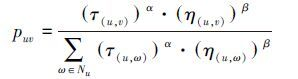1.3 信息素更新机制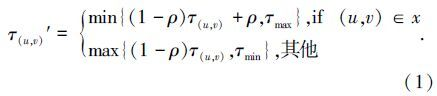2 理论分析方法及数学工具

2.1 适应值划分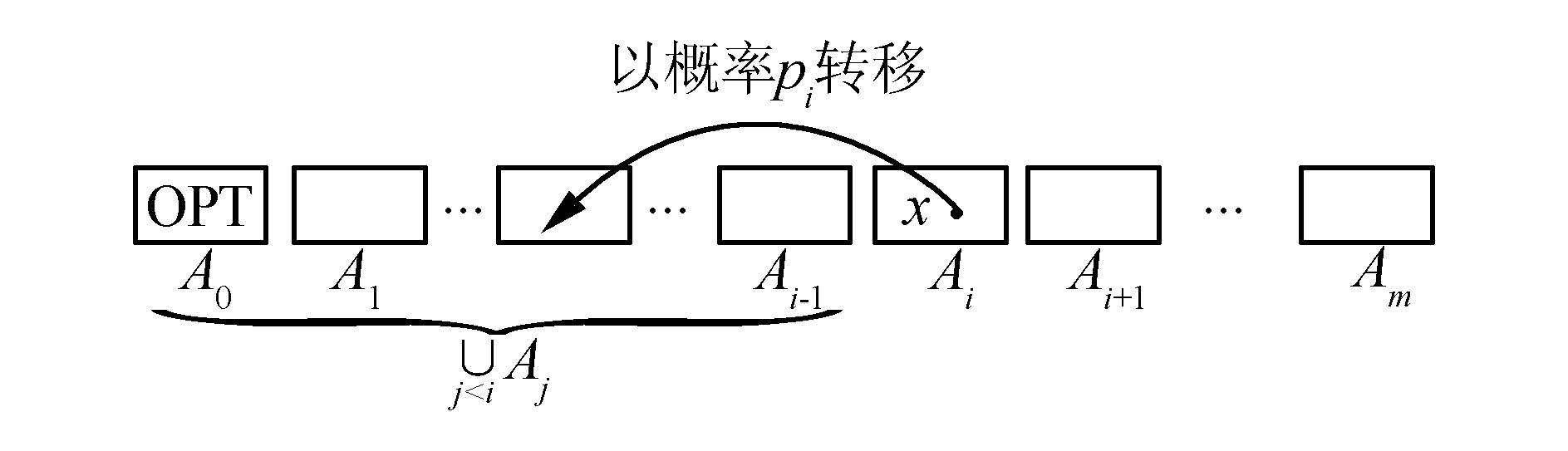图 1 适应值划分 Fig. 1 Fitness values partition

2.2 期望倍增距离减少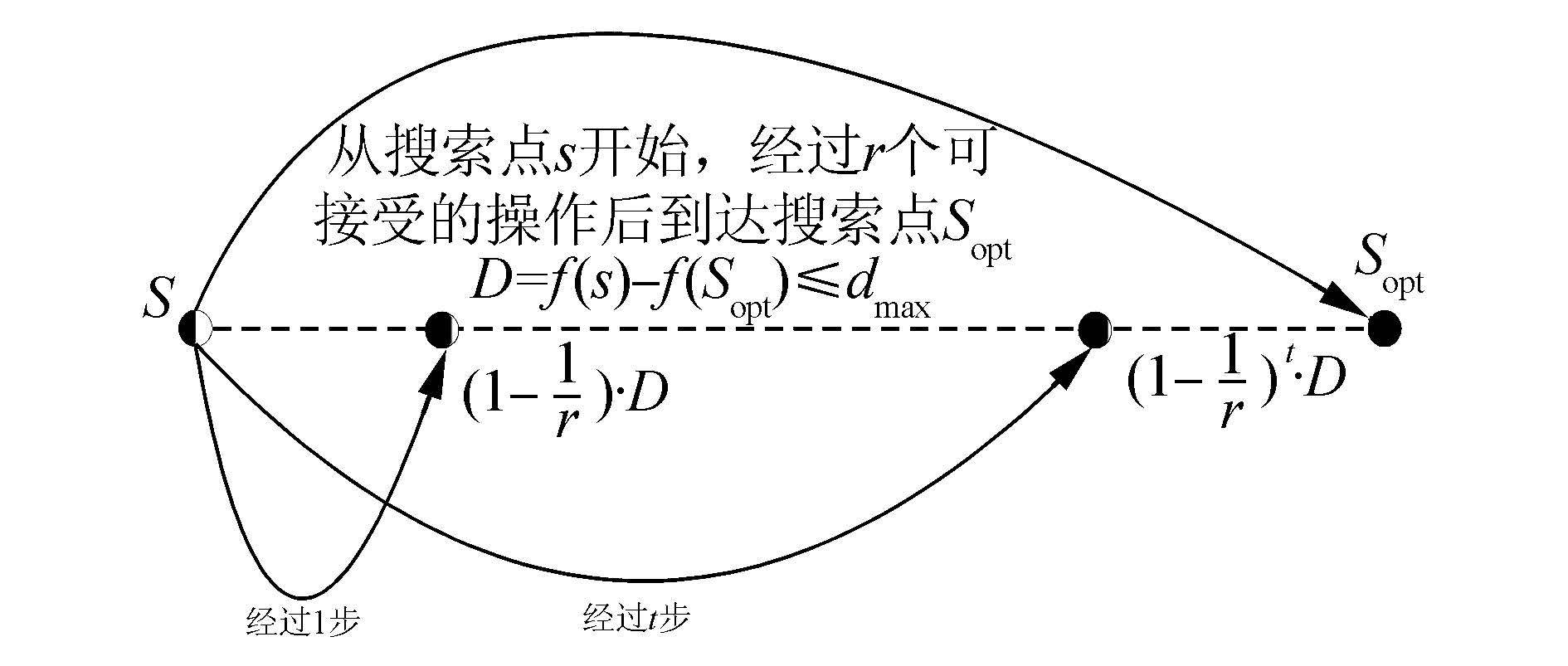图 2 期望倍增距离减少 Fig. 2 Expected multiplicative distance decrease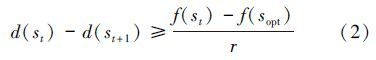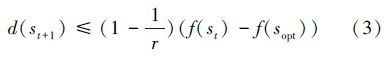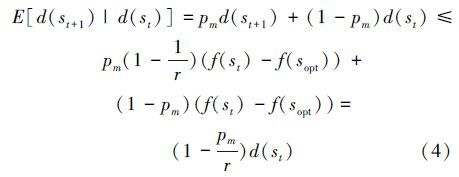Yt=d(st),有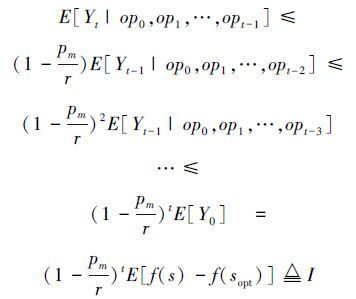T=O(r·logD),当tT,有E[Yt|op0,op1,…,opt－1]≤(1－${\alpha \over r}$)tD≤${1 \over 2}$。根据Markov不等式,算法执行T步之后,离最优解距离至少为1的概率P(Yt≥1)≤${1 \over 2}$因此P(Yt<1)≥${1 \over 2}$。也就是说T步之后与目标最优解距离小于1的概率至少为1/2。 假设函数适应值均为正整数,则算法获得最优解(离目标最优解距离为0)的概率至少为1/2。因此,算法最终到达目标找到最优解的期望时间上界为2T=O(r·logD)。

2.3 尾概率不等式

2.3.1 Markov不等式

2.3.2 Chebyshev不等式

1)令X为一随机变量,其中E(X)=μ, Va(X)=σ2。对于k>0,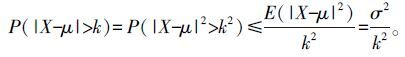2)令Xi~iid(independent and identically distributed),独立同分布，期望E(Xi)=μ 且Va(Xi)=σ2，${\bar X}$为μ的估计,则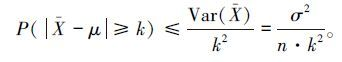2.3.3 Chernoff界

3 一些理论研究结果及问题讨论 3.1 参数ρ、α、β对算法性能影响

Neumann[27, 29]等研究了信息素挥发因子 ρ对蚁群算法性能的影响,指出如果ρ≥${{n - 2} \over {3n - 2}}$,即当n→∞,ρ≥1/3时,算法 1-ANT的行为类似于(1+1)ANT,就是说,1-ANT在每个函数上的期望运行时间和(1+1)ANT相同。同时指出,如果取 ρ=O(n－1－ε),ε>0为常数,则算法 1-ANT以1－2Ω(nε/3)的概率求解 OneMax函数的时间为2Ω(nε/3)；如果取ρ=Ω(n－1+ε),ε>0为常数,则算法1-ANT以1－2Ω(nε/2)的概率求解 OneMax函数的时间为O(n2),给出了 1-ANT算法求解OneMax函数的时间复杂度从指数时间到多项式时间的相变现象。Doerr等使用一个信息素模型简化了文献的分析,进一步研究了挥发因子ρ∈[1/n1+ε,1/n1－ε]的优化时间。并指出,存在常数c∈(0,1)和NN,使得当nNρc,对于n个变元的OneMax函数,算法1-ANT以小于1/e${c \over \rho }$的概率优化时间最多为e${c \over \rho }$Doerr[32, 33]研究了挥发因子ρ对于1-ANT算法的性能影响,指出对于LeadingOnes和BinVal两个函数,存在一个相变现象,且阈值为ρ~1/(nlogn),其期望时间为指数级到多项式。

3.2 从1-ANT到n-ANT

Neumann等人研究了λ-MMASIB算法求解拟布尔函数的性能。他们指出,当 λ=2,也即构造2个蚂蚁解时,该算法在挥发因子足够小的情况下,能够在多项式时间内求解OneMax函数。

Attiratanasunthron和Fakcharoenphol研究了ACO算法求解有向无环图单源最短路径问题的多项式时间界。特别地,对于顶点数为n，边数为m的有向无环图,具有n只蚂蚁的n-ANT算法能够在期望时间O(n2mlogn/ρ)内求解单源最短路径问题。

3.3 从拟布尔函数到NP难问题 3.3.1 拟布尔函数

1)OneMax(x)=$\sum\limits_{i = 1}^n {{x_i}}$;

2)LeadingOnes(x)=$\sum\limits_{i = 1}^n {\prod\limits_{j = 1}^i {{x_i}} }$;

3)Trap(x)=$\sum\limits_{i = 1}^n {{x_i}}$+(n+1)·$\prod\limits_{i = 1}^n {}$(1－xi);

4)BinVal(x)=$\sum\limits_{i = 1}^n {{2^{n - i}}} {x_i}$;

5)Needle(x)=$\prod\limits_{i = 1}^n {{x_i}}$;

6)NH-OneMax(x)=($\prod\limits_{i = 1}^k {{x_i}}$)=($\sum\limits_{i = k + 1}^n {{x_i}}$);

7) F(x)= $\sum\limits_{i = 1}^n {{w_i}} {x_i}$。

3.3.2 P问题

3.3.3 NP难问题

 问题 说明 算法模型 时间复杂度 相关文献 OneMax OneMax(x)=$\sum\limits_{i = 1}^n {{x_i}}$ 1-ANT O(nlogn) Neumann [27, 29] LeadingOnes LeadingOnes(x)=$\sum\limits_{i = 1}^n {\prod\limits_{j = 1}^i {{x_i}} }$ MMAS* O(n2+(nlogn)/ρ) Neumann等 Linear function F(x)= $\sum\limits_{i = 1}^n {{w_i}} {x_i}$i MMAS/ MMAS* O((n3logn)/ρ) Kötzing等 Minimum spanning tree 最小生成树问题 1-ANT O(mn(logn+logwmax)) Neumann 和 Witt  Minimum cut problem 最小割问题(带权图);信息素的界为[l,h],cn=${h \over l}$, MMAS* O(n2)(α=0,β=1)； Kötzing 等 $O\left( {{{\left( {n - 2 + 2{c_n}} \right)!} \over {\left( {n - 2} \right)!\left( {2{c_n}} \right)!}}} \right)$ 若cn=k为常数, O(n2k)(α=1,β=1)； Single Destination Shortest Path, SDSP 其中m为给定图的边数,Δ为最大出度,l为所有最短路径(如果不止一条)的最大边数,ρ为ACO算法的挥发因子 n-ANT O(${{m\Delta l\log \left( {\Delta l} \right)} \over \rho }$) Attiratanasunthron等 All-pairs Shortest Path(APSP) MMASAPSP O(Δll*+l/ρ) Horoba和Sudholt  Dynamic SDSP λ-MMAS O(l+lln(τmax/τmin)/ρ)(λ= 2e/τmin) Lissovoi和Witt TSP 完全图实例 (1+1)MMAA O(n6+(1/ρ)nlnn)(α=1,β= 0)；O(n5+(1/ρ)nlnn)(α=1,β=1) Zhou TSP G1 MMASArb* O(n3logn+nlogn/ρ)(α=1,β=1) Kötzing等

4 结束语

1)蚁群算法理论分析工具还非常有限，这也是阻碍其向前发展的一个主要因素。现有的理论分析方法主要是马尔可夫链理论、适应值划分技术及漂移分析等方法。这些工具或者本身比较复杂，或者只是适应一些特殊情形的分析，甚至还需要满足一些严格的条件才能使用。因此寻求新的方法和工具也是未来的一个方向。

2)当前蚁群优化算法理论研究局限于一些简化的算法模型，基于种群的、更加复杂的构造过程还有待于进一步深入研究。对于n-ANT算法的有效性如何，是否蚂蚁的数量与算法的时间复杂度存在某种关系还未知。通过研究群体规模、构造过程、算法参数等对算法性能的影响，深入挖掘蚁群优化算法的潜能，更好地指导算法设计及应用。对于一个NP难解组合优化问题，蚁群算法能否获得比已有算法更好的近似比？如何获得更紧的时间界等，这些问题都有待于深入研究。

3)将现有蚁群优化算法的理论分析结果扩展到更多的智能算法中。目前在差分进化算法、分布评估算法、粒子群算法以及Memetic算法等随机启发式搜索的理论研究还非常有限，可以尝试将现有的理论分析结论进行有效扩展。此外，当前蚁群算法主要关注离散空间优化，可以向连续优化做进一步扩充。通过对更多算法、更多问题的研究，从中找出一些共性的东西，进一步丰富和增强群智能搜索算法的理论基础。

  DORIGO M, MANIEZZO V, COLORNI A. Theantsystem:An autocatalytic optimizing process Technical Report 91-016[R]. Milan, Italy:Dipartimento di Elettronica, Politecnico di Milano, 1991.  DORIGO M, STUTZLE T. 蚁群优化[M]. 张军, 胡晓敏, 罗旭耀, 译. 北京:清华大学出版社, 2007:110-246.  DORIGO M, BLUM C. Ant colony optimization theory:a survey[J]. Theoretical computer science, 2005, 344(2-3):243-278.  段海滨, 王道波, 于秀芬. 蚁群算法的研究现状及其展望[J]. 中国工程科学, 2007, 9(2):98-102.DUAN Haibin, WANG Daobo, YU Xiufen. Ant colony algorithm:survey and prospect[J]. Engineering science, 2007, 9(2):98-102.  NOCEDAL J, WRIGHT S J. Numerical optimization[M]. New York:Springer, 2000.  VAZIRANI V V. Approximation algorithms[M]. Berlin Heidelberg:Springer, 2003.  GAREY M R, JOHNSON D S. Computers and Intractability-A Guide to the Theory of NP-Completeness[M]. New York:Freeman W H and Company, 1979.  COLORNI A, DORIGO M, MANIEZZO V. An investigation of some properties of an "Ant algorithm"[C]//Proceedings of Parallel Problem Solving from Nature II (PPSN 92). Brussels, Belgium, 1992:515-526.  STVTZLE T, HOOS H H. MAX-MIN ant system[J]. Future generation computer systems, 2000, 16(8):889-914.  GUTJAHR W J. Mathematical runtime analysis of ACO algorithms:survey on an emerging issue[J]. Swarm intelligence, 2007, 1(1):59-79.  DROSTE S, JANSEN T, WEGENER I. On the analysis of the (1+1) evolutionary algorithm[J]. Theoretical computer science, 2002, 276(1-2):51-81.  WEGENER I, WITT C. On the analysis of a simple evolutionary algorithm on quadratic pseudo-boolean functions[J]. Journal of discrete algorithms, 2005, 3(1):61-78.  WEGENER I. Towards a theory of randomized search heuristics[M]//ROVAN B, VOJTÁŠ P. Mathematical Foundations of computer science 2003. Berlin Heidelberg:Springer, 2003:125-141.  WEGENER I. Methods for the analysis of evolutionary algorithms on pseudo-boolean functions[M]//Evolutionary Optimization. Dordrecht:Kluwer Academic Publishers, 2002, 48:349-369.  BEYER H G, SCHWEFEL H P, WEGENER I. How to analyse evolutionary algorithms[J]. Theoretical computer science, 2002, 287(1):101-130.  HE J, YAO X. Towards an analytic framework for analysing the computation time of evolutionary algorithms[J]. Artificial intelligence, 2003, 145(1-2):59-97.  HE J, YAO X. Drift analysis and average time complexity of evolutionary algorithms[J]. Artificial intelligence, 2001, 127(1):57-85.  HE J, YAO X. From an individual to a population:an analysis of the first hitting time of population-based evolutionary algorithms[J]. IEEE transactions on evolutionary computation, 2002, 6(5):495-511.  GUTJAHR W J. A graph-based ant system and its convergence[J]. Future generation computer systems, 2000, 16(8):873-888.  STVTZLE T, DORIGO M. A short convergence proof for a class of ACO algorithms[J]. IEEE transactions on evolutionary computation, 2002, 6(4):358-365.  GUTJAHR W J. On the finite-time dynamics of ant colony optimization[J]. Methodology and computing in applied probability, 2006, 8(1):105-133.  黄翰, 郝志峰, 吴春国,等. 蚁群算法的收敛速度分析[J]. 计算机学报, 2007, 30(8):1344-1353. HUANG Han, HAO Zhifeng, WU Chunguo, et al. The convergence speed of ant colony optimization[J]. Chinese journal of computers, 2007, 30(8):1344-1353.  BIRATTARI M, Dicaro G, DORIGO M. Toward the formal foundation of ant programming[M]//DORIGO M, DI CARO G, SAMPELS M. Ant Algorithms. Berlin Heidelberg:Springer-Verlag, 2002, 2463:188-201.  MEULEAU N, DORIGO M. Ant colony optimization and stochastic gradient descent[J]. Artificial life, 2002, 8(2):103-121.  ZLOCHIN M, BIRATTARI M, MEULEAU N, et al. Model-based search for combinatorial optimization:a critical survey[J]. Annals of operations research, 2004, 131(1-4):373-395.  GUTJAHR W J. A generalized convergence result for the graph-based ant system metaheuristic[J]. Probability in the engineering and informational sciences, 2003, 17(4):545-569.  NEUMANN F, WITT C. Runtime analysis of a simple ant colony optimization algorithm[C]//Proceedings of the 17th International Symposium on Algorithms, ISAAC 2006. Kolkata, India, 2006, 4288:618-627.  NEUMANN F, SUDHOLT D, Wit C. Comparing variants of MMAS ACO algorithms on pseudo-Boolean functions[C]//Proceedings of International Workshop, SLS 2007. Brussels, Belgium, 2007:61-75.  NEUMANN F, WITT C. Runtime analysis of a simple ant colony optimization algorithm[J]. Algorithmica, 2009, 54(2):243-255.  GUTJAHR W J. First steps to the runtime complexity analysis of ant colony optimization[J]. Computers & operations research, 2008, 35(9):2711-2727.  DOERR B, JOHANNSEN D. Refined runtime analysis of a basic ant colony optimization algorithm[C]//Proceedings of the IEEE Congress on Evolutionary Computation (CEC 2007). Singapore, 2007:501-507.  DOERR B, NEUMANN F, SUDHOLT D, et al. On the runtime analysis of the 1-ANT ACO algorithm[C]//Proceedings of Genetic and Evolutionary Computation Conference (GECCO'2007). London, England, 2007:33-40.  DOERR B, NEUMANN F, SUDHOL D, et al. Runtime analysis of the 1-ANT ant colony optimizer[J]. Theoretical computer science, 2011, 412(17):1629-1644.  GUTJAHR W J, SEBASTIANI G. Runtime analysis of ant colony optimization with best-so-far reinforcement[J]. Methodology and computing in applied probability, 2008, 10(3):409-433.  NEUMANN F, SUDHOLT D, WITT C. Analysis of different MMAS ACO algorithms on unimodal functions and plateaus[J]. Swarm intelligence, 2009, 3(1):35-68.  NEUMANN F, SUDHOLT D, WITT C. A few ants are enough:ACO with Iteration-best update[C]//Proceedings of Genetic and Evolutionary Computation Conference (GECCO'2010). Portland, USA, 2010:63-70.  KÖTZING T, NEUMANN F, SUDHOLT D, et al. Simple Max-Min ant systems and the optimization of linear pseudo-Boolean functions[C]//Proceedings of the 11th Work-shop on Foundations of Genetic Algorithms (FOGA 2011). New York, NY, USA, 2011:209-218.  NEUMANN F, SUDHOLT D, WITT C. Rigorous analyses for the combination of ant colony optimization and local search[C]//Proceedings of the 6th International Conference on Ant Colony Optimization and Swarm Intelligence (ANTS08). Brussels, Belgium, 2008:132-143.  NEUMANN F, WITT C. Ant colony optimization and the minimum spanning tree problem[J]. Theoretical computer science, 2010, 411(25):2406-2413.  KÖTZING T, LEHRE P K, OLIVETO P S, et al. Ant colony optimization and the minimum cut problem[C]//Proceedings of the 12th Annual Conference on Genetic and Evolutionary Computation Conference (GECCO'10). New York, NY, USA, 2010:1393-1400.  ATTIRATANASUNTHRON N, FAKCHAROENPHOL J. A running time analysis of an ant colony optimization algorithm for shortest paths in directed acyclic graphs[J]. Information processing letters, 2008, 105(3):88-92.  SUDHOLT D, THYSSEN C. Running time analysis of Ant Colony Optimization for shortest path problems[J]. Journal of discrete algorithms, 2012, 10:165-180.  SUDHOLT D, THYSSEN C. A simple ant colony optimizer for stochastic shortest path problems[J]. Algorithmica, 2012, 64(4):643-672.  LISSOVOI A, WITT C. Runtime analysis of ant colony optimization on dynamic shortest path problems[J]. Theoretical computer science, 2015, 561:73-85.  ZHOU Y R. Runtime analysis of an ant colony optimization algorithm for TSP instances[J]. IEEE transactions on evolutionary computation, 2009, 13(5):1083-1092.  KÖTZING T, NEUMANN F, RÖGLIN H, et al. Theoretical analysis of two ACO approaches for the traveling sales man problem[J]. Swarm intelligence, 2012, 6(1):1-21.  LISSOVOI A, WITT C. MMAS versus population-based EA on a family of dynamic fitness functions[J]. Algorithmica, 2015, doi:10.1007/s00453-015-9975-z.
DOI: 10.11992/tis.201510002

0

#### 文章信息

XIA Xiaoyun, ZHOU Yuren

Advances in theoretical research of ant colony optimization

CAAI Transactions on Intelligent Systems, 2016, 11(01): 27-36.
DOI: 10.11992/tis.201510002